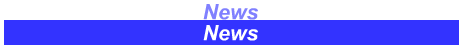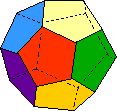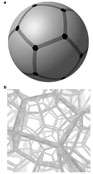A Dodecahedral Universe?

Alec MacAndrew

I have written an essay on modern cosmology which is here. The essay touches on the significance of the cosmic microwave background (CMB) anisotropy and on other observational evidence for the Big Bang

If you read this essay you will have noticed this intriguing statement:

In fact the lack of power in the angular spectrum at very large scales, first seen in COBE and confirmed in WMAP, (certainly at the quadrupole, l = 2, and to some extent at the octopole) has been remarked on by a number of observers (22).  However for multipole l › 3, agreement with the standard model is remarkable.  (The Integrated Sachs-Wolfe effect predicts enhanced power at low values of the multipole and WMAP and COBE data trend in the opposite sense….’

This is something of a puzzle, as such a good match across the spectrum between theory and observations is spoiled by unexplained data at large angular scales.

What this means is that the minute ripples in the microwave background (the 2.7° Kelvin radiation echo from the Big Bang) tell us a huge amount about the early universe.  The essay explains some of this, but suffice to say that ripples occur at a wide range of angular scales – there are very fine ripples and very broad ripples (we call it ‘scale-invariant’). The WMAP and COBE measurements have a puzzling lack of ‘rippliness’ on the biggest scales – ripples which cover dimensions of 60° to 90° in the sky (which is the angular scale of the l = 2 quadrupole) or about 16 billion light years across on the surface of last scattering (the spherical surface 13.7 billion light years away from which the microwave background that is currently observed arose)

An intriguing paper in Nature puts forward an hypothesis for this loss of power at large angular scales (1) The hypothesis is that the universe is finite and closed and has the spatial topology of a Poincaré spherical dodecahedron with opposite faces abstractly glued together so that as you exit or look out of one face you enter or look into the opposite face.

Go here to see what a Euclidean dodecahedron looks like (2):  http://mathworld.wolfram.com/Dodecahedron.html[A spherical dodecahedron is to a Euclidean dodecahedron (like the one illustrated above) as a spherical pentagon (12 of which when fitted together form a sphere) is to a plane pentagon.  In the same way as 12 spherical pentagons fit together perfectly to make a sphere, which is the surface of a 3-D ball, 120 spherical dodecahedrons fit together perfectly to make a hypersphere which is the 3-D surface of a 4-D ball].Spherical pentagons tile a sphere; spherical dodecahedrons tile a hypersphere (After ref 1)

Because such a universe would be finite and closed it could not sustain the largest scale ripples in the microwave background.  The size of the biggest ripples would be constrained by the finite size of the universe.  This would explain the lack of power in the low multipoles of the CMB.  There are many possible finite topologies for the universe – this dodecahedral one predicts the relative 'rippliness’ of the microwave background at the biggest scales of the dipole, the quadrupole and the octopole (l=1, l=2 and l=3) very well.

This is only a hypothesis at the moment and it presents some problems – inflation for example is well supported by other observations and explains the homogeneity of the universe and the fact that it is flat or nearly so – it is not clear whether an inflationary epoch can be supported in models that result in a ‘small’ universe.

There are ways to test this hypothesis experimentally: first it predicts Ω0 ≈  1.013 > 1 (Ω0 = 1 for a flat, infinite universe).  If Ω0 is found to be less than 1.01 the dodecahedral hypothesis is disproven. (WMAP measures Ω0 = 1.02 but is insufficiently accurate to rule out Ω0 < 1.01; and the new satellite experiment, Planck, will give a more accurate measurement).  Second, interconnected space topologies such as this would produce correlations of temperature on matching circles in the sky (3).  None have been found in WMAP data so far, but a very sensitive measurement would be required, to remove other artefacts and noise.

It will be interesting to see whether future data will support this hypothesis.  If it does, we might find we live in a universe that is finite and ‘only’ a few billion or tens of billions of light years across.

1) Luminet et al, Dodecahedral space topology as an explanation for weak wide-angle temperature correlations in the cosmic microwave background, Nature 425, 593 – 595.

2) Go here to see a dodecahedron and find out some interesting facts about it:

http://mathworld.wolfram.com/Dodecahedron.html

3) Cornish et al, Circles in the sky: Finding topology with microwave background radiation, Class Quant Grav 15, 2657 -2670